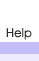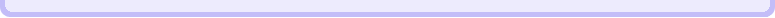Worksheet Creators Need to generate random worksheets on the fly? Look no further. The Worksheet Creators by Brainormous are so loaded with features, you'll never be at a loss for a new worksheet for standard arithmetic again. Answer keys with an ID code for easy organization Random problem generation Editable problems; if you don't like some of the randomly generated problems, simply click on the problem and edit the numbers Graphics (Subtraction and Addition only; color or blackline to save ink) Horizontal and Vertical orientations Random and Ordered problem layout Up to 30 problems per page (24 problems for Division) Up to 3 digit decimals on Division problems Show remainders for Division problems Reverse problem solving (Find the second number in the problem when the answer is given Advanced problems: up to 4 digit numbers (3 digit for Multiplication) Subtraction problems with negative number answers Option to display a numberline at the bottom of the page (Addition and Subtraction only) Option to round the first and/or second number in the problem Option for increased font size You can use the Worksheet Creator demos online (up to 10 problems for Addition, Subtraction, and Multiplication, and 8 problems for Division), or purchase a downloadable version to play offline. Online: